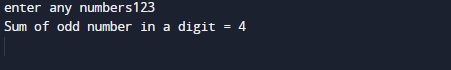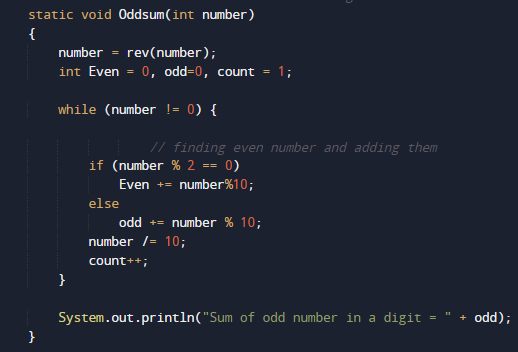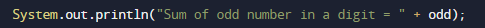# Java Program to Find Sum of odd digits in a number

In this tutorial you will learn about the Java Program to Find Sum of odd digits in a number and its application with practical example.

In this tutorial, we will learn to create a Java Program to Find sum of odd digits in a number using Java programming.

## Prerequisites

Before starting with this tutorial we assume that you are best aware of the following Java programming topics:

• Java Operators.
• Basic Input and Output function in Java.
• Class and Object in Java.
• Basic Java programming.
• If-else statements in Java.
• For loop in Java.
• Built in functions.

## What is Even number.

Even number are that number  that can be divided by two and gives remainder equals to zero and ends with 2,4,6,8 and with 0.
Example: (No/2==0) =>  (8/2==0) so 8 is a even Number.
Some even numbers are 2, 4, 6, 8, 10.

## What is Odd Number

Odd numbers are number that are not divide by 2  and does not give remainder to Zero, when divided by 2, some the odd numbers are end with 1, 3, 5, 7, 9.

Example: (No/2==0) =>  (5/2==1) so 5 is an Odd Number.

## Java Program to Find Sum of odd digits in a number.

In this program we will learn to create a program that will find sum of Odd digits in a given  number. Firstly we declare required variable and also initiates required values in variable. Next we take value from user at run time and then after we will find the sum of Odd digits in a given number.

## OutputIn the above program, we have first declared and initialized a set variables required in the program.

• number= it will hold entered number.
• Even = it will hold sum or even digit.
• rev = for reversing a string.Here in our program we first declared and initialized set of  required variables in the program.Then take a value from user at run time.Afterword we pass the given number to function named Oddsum(). where we calculate sum of all odd digit of a  given number.Here we create a method named Oddsum() for finding all the odd number in a digit and adding them at the end return sum of all odd values and print the required output..

In this tutorial we have learn about the Java Program to Find Sum of odd digits in a number and its application with practical example. I hope you will like this tutorial.